# BT PQ P1-T2-20-16-2: Univariate regression: Portfolio versus benchmark returns

Simulated portfolio & benchmark for purposes of testing basic features of univariate regression

David Harper https://www.bionicturtle.com/
2022-01-05

20.16.2. Peter is an analyst who is evaluating an investment fund whose managers claim has outperformed their benchmark. He collected monthly returns for the last five years; i.e., the sample size is excess return pairs over n = 60 months. He plots excess returns, which are defined as the returns in excess of the riskfree rate; ie., an excess return equals the gross return minus the riskfree rate. The scatterplot is displayed below:

scatterplot

The correlation coefficient is 0.708. In regard to the univariate data, the standard deviation of the portfolio’s returns is 22.84% and the standard deviation of the benchmark’s returns is 9.79%. The average excess return of the benchmark was -0.37% and the average excess return of the portfolio was 2.61%. Each of the following statements is true EXCEPT which is false?

1. The slope of the regression line is approximately 1.65 and the intercept is approximately 3.22%
2. Visual inspection confirms the error variance is not constant and we can, therefore, assert the presence of heteroskedastic shocks
3. This regression line passes through the coordinates of averages, (μ_x, μ_y) = (-0.37%, +2.61%), although this is not an actual pairwise observation
4. This model appears to at least meet the three essential restrictions of a linear regression model including linearity in the coefficients (aka, parameters)
``````library(tidyverse)
library(scales)
library(ggthemes)
# library(lmtest)

x_mu <- .01; x_sig <- .1

y_mu <- .03; y_sig <- .2

rho <-  0.72
months <- 60

#set.seed(59)
set.seed(158)

# 60  rows of random standard normals
returns <- tibble(index = 1:months)
returns\$x1 <- rnorm(months)
returns\$y1 <- rnorm(months)

# make y2 correlated with y1; adjust location/scale
returns1 <- returns %>% mutate(
y2 = rho*x1 + sqrt(1 - rho^2)*y1,
r_x = x_mu + x1 * x_sig,
r_y = y_mu + y2 * y_sig
)

x_sd <- sd(returns1\$r_x)
y_sd <- sd(returns1\$r_y)
rho_xy <- cor(returns1\$r_x, returns1\$r_y)
beta_yx <- rho_xy * y_sd / x_sd
x_mu_act <- mean(returns1\$r_x)
y_mu_act <- mean(returns1\$r_y)

sprintf("sample rho is %.4f. The standard deviation of Portfolio returns is ", rho_xy)
``````
`` "sample rho is 0.7078. The standard deviation of Portfolio returns is "``
``````paste("Portfolio standard deviation is", percent(y_sd, accuracy = .01))
``````
`` "Portfolio standard deviation is 22.84%"``
``````paste("Benchmark standard deviation is", percent(x_sd, accuracy = .01))
``````
`` "Benchmark standard deviation is 9.79%"``
``````paste("Portfolio average excess return is", percent(y_mu_act, accuracy = .01))
``````
`` "Portfolio average excess return is 2.61%"``
``````paste("Benchmark average excess return is", percent(x_mu_act, accuracy = .01))
``````
`` "Benchmark average excess return is -0.37%"``
``````sprintf("Beta(P,B) is %.3f", beta_yx)
``````
`` "Beta(P,B) is 1.652"``
``````returns1_lm <- lm(r_y ~ r_x, data = returns1)
summary(returns1_lm)
``````
``````
Call:
lm(formula = r_y ~ r_x, data = returns1)

Residuals:
Min       1Q   Median       3Q      Max
-0.30810 -0.11231 -0.01385  0.09922  0.42134

Coefficients:
Estimate Std. Error t value Pr(>|t|)
(Intercept)  0.03231    0.02102   1.537     0.13
r_x          1.65219    0.21649   7.632 2.54e-10 ***
---
Signif. codes:  0 '***' 0.001 '**' 0.01 '*' 0.05 '.' 0.1 ' ' 1

Residual standard error: 0.1627 on 58 degrees of freedom
Multiple R-squared:  0.501, Adjusted R-squared:  0.4924
F-statistic: 58.24 on 1 and 58 DF,  p-value: 2.543e-10``````
``````returns1 %>% ggplot(aes(x = r_x, y = r_y)) +
geom_point() +
geom_smooth(method = "lm", se = FALSE, color = "forestgreen", linetype = "longdash", size = 1.5) +
ggtitle("Investment fund versus benchmark: Excess returns, n = 60 months") +
xlab("Benchmark's excess returns") +
ylab("Portfolio's excess returns") +
scale_x_continuous(labels = percent_format(accuracy = 1)) +
scale_y_continuous(labels = percent_format(accuracy = 1)) +
theme_light() +
theme(
axis.title.y = element_text(size = 14),
axis.title.x = element_text(size = 14),
axis.text.x = element_text(size = 14, margin = margin(b = 10)),
axis.text.y = element_text(size = 14, margin = margin(l = 10))
)
``````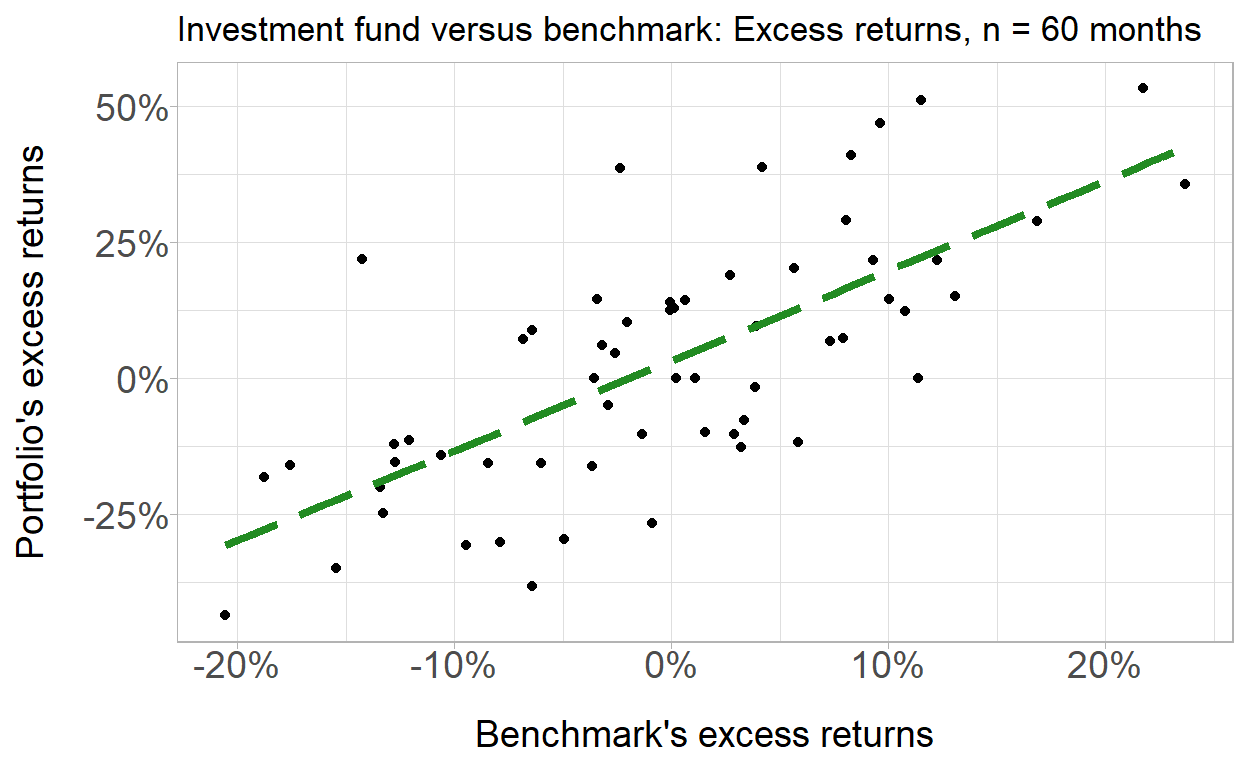``````plot(returns1_lm)
``````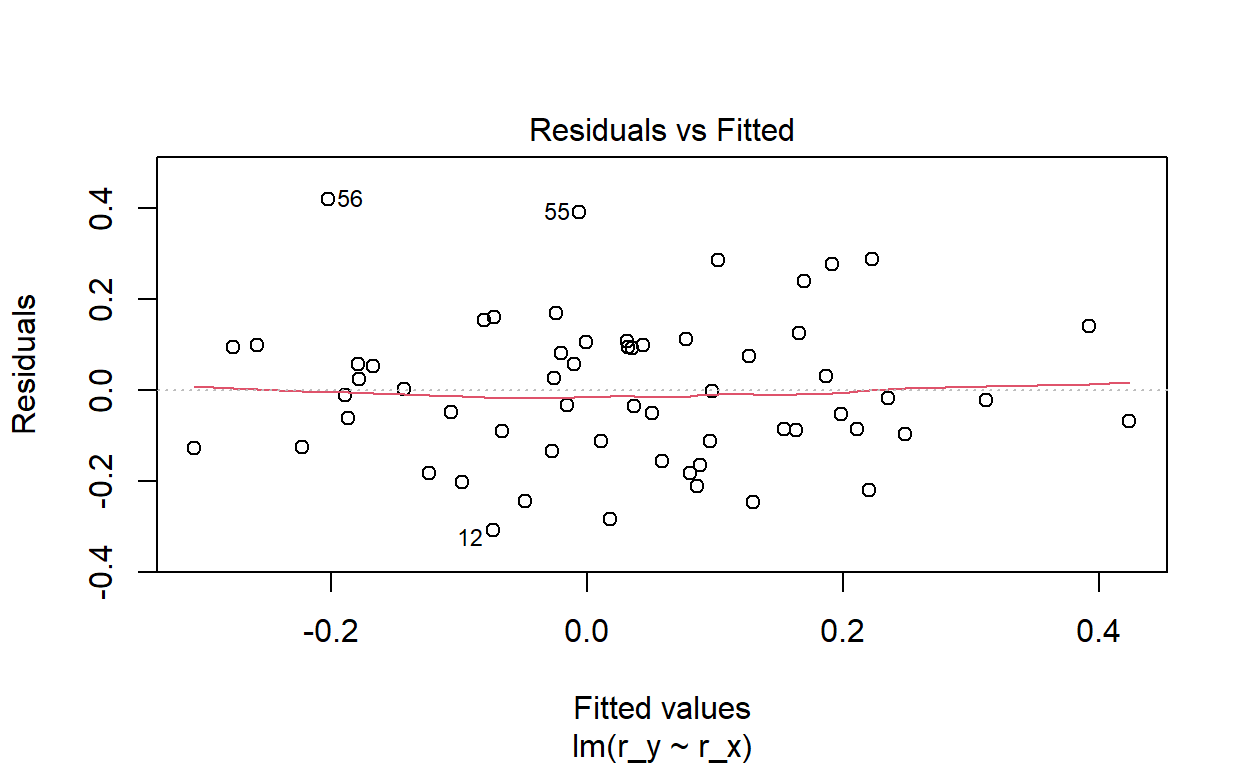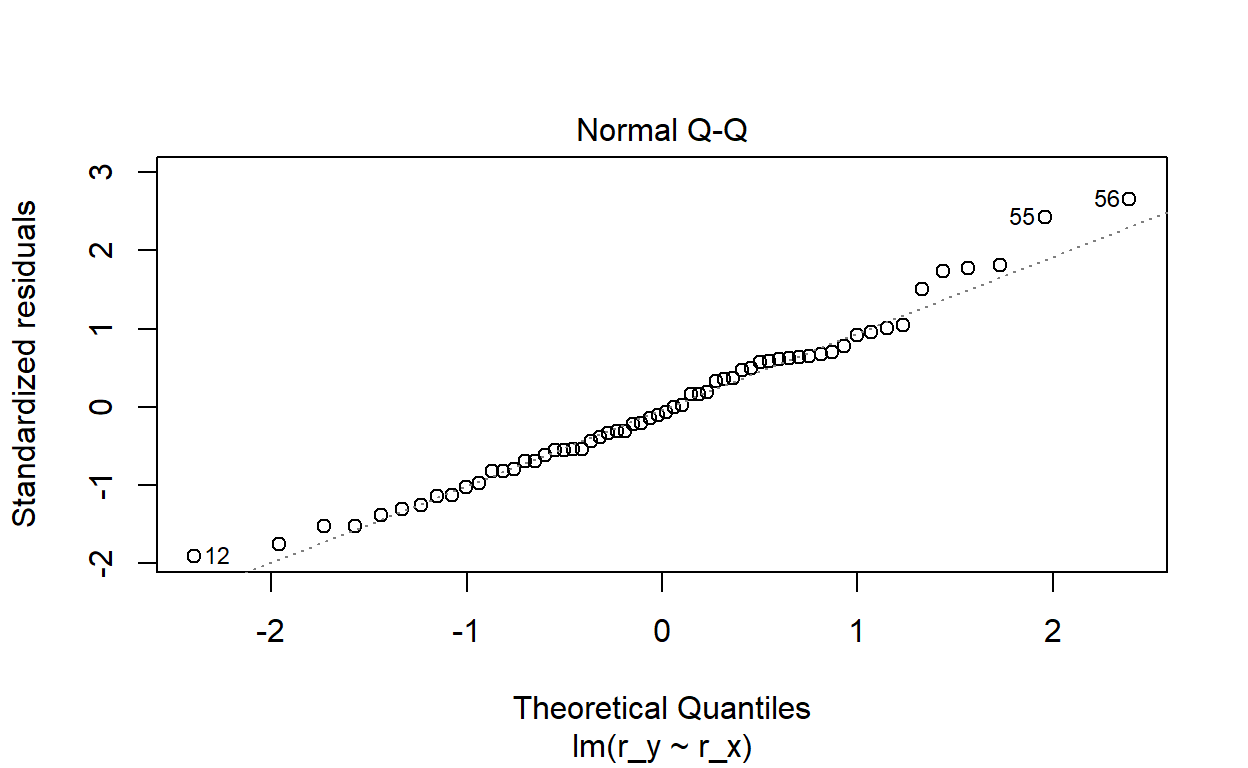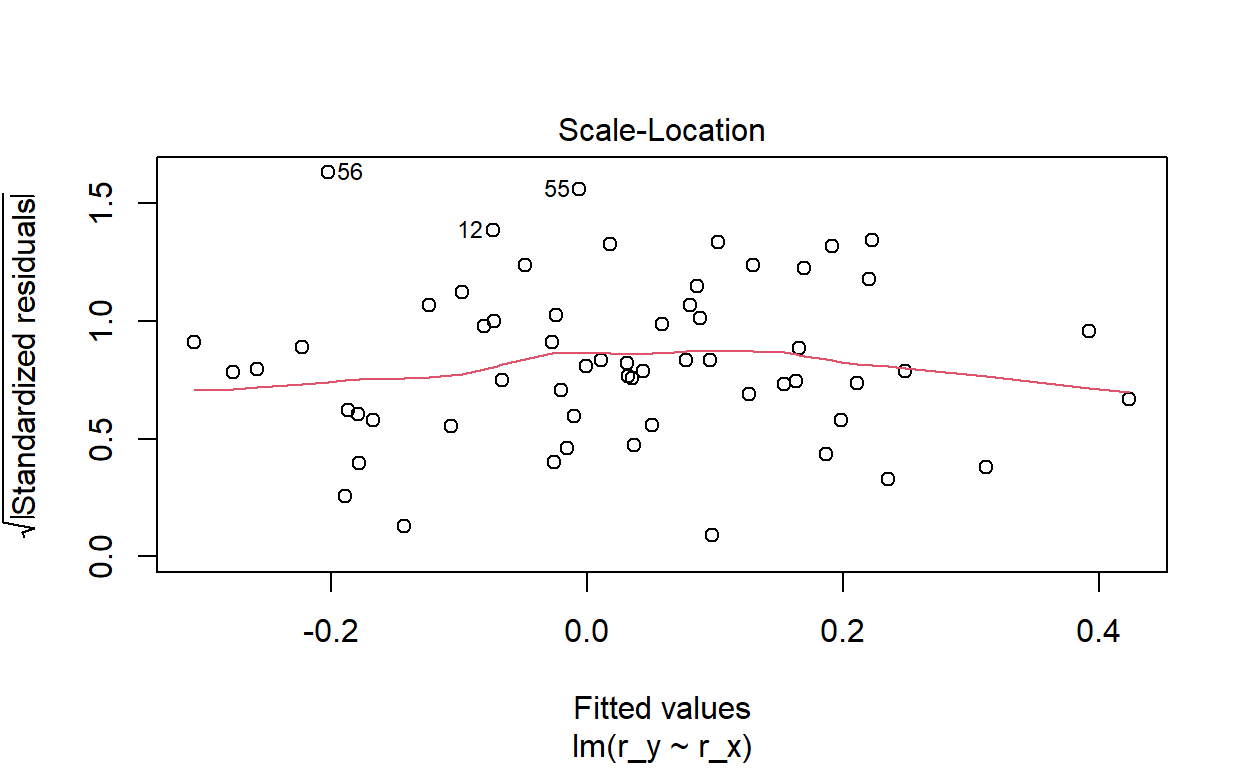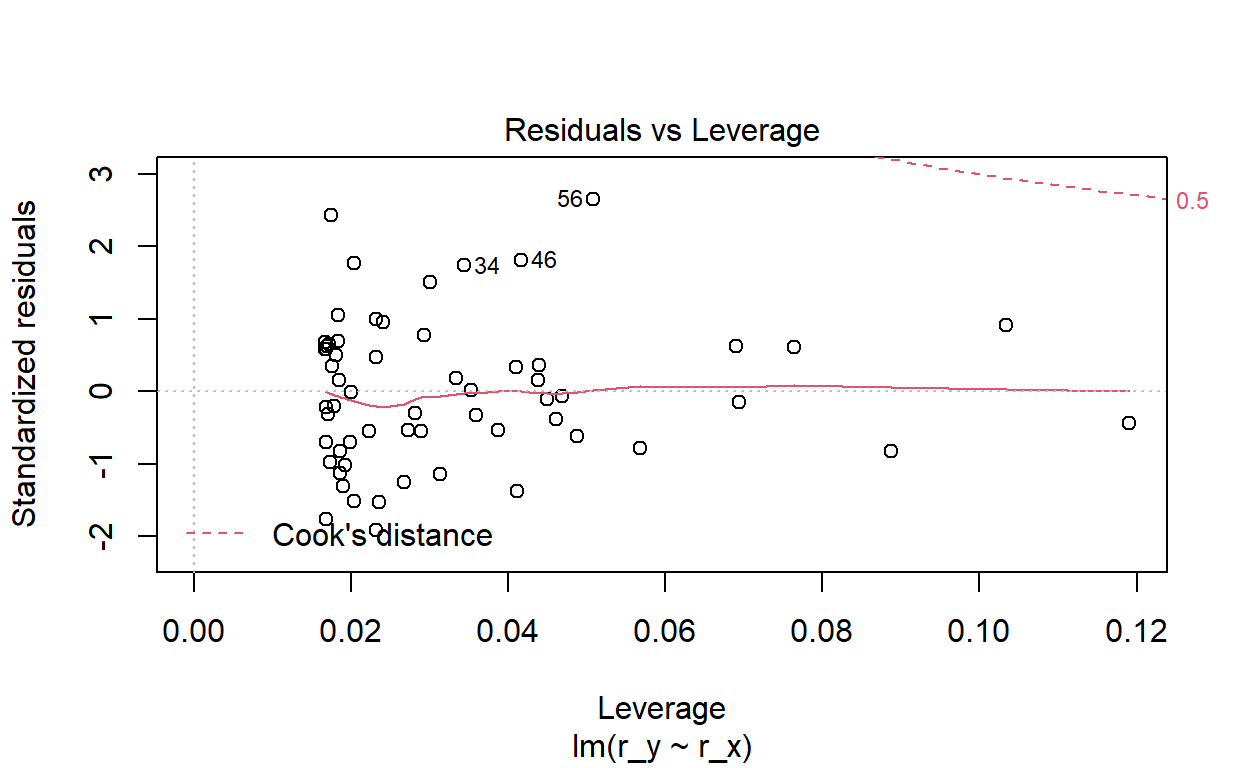``````new.df <- data.frame(r_x = c(x_mu_act, 0, seq(from = 0.01, to = 0.1, by = .01)))
new.df\$predicted_y <- predict(returns1_lm, new.df)
new.df
``````
``````            r_x predicted_y
1  -0.003740684  0.02613168
2   0.000000000  0.03231199
3   0.010000000  0.04883388
4   0.020000000  0.06535577
5   0.030000000  0.08187766
6   0.040000000  0.09839955
7   0.050000000  0.11492143
8   0.060000000  0.13144332
9   0.070000000  0.14796521
10  0.080000000  0.16448710
11  0.090000000  0.18100899
12  0.100000000  0.19753088``````
``````intercept_predict <- (0 - x_mu_act)*beta_yx + y_mu_act
intercept_predict_round <- (0 - round(x_mu_act,4))*beta_yx + round(y_mu_act,4)
intercept_predict
``````
`` 0.03231199``
``````intercept_predict_round
``````
`` 0.0322131``